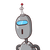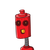# prove that if chords of congruent circles subtend to equal angles at the centre then the chords are equal​

prove that if chords of congruent circles subtend to equal angles at the centre then the chords are equal​

### 2 thoughts on “prove that if chords of congruent circles subtend to equal angles at the centre then the chords are equal​”

1.Step-by-step explanation:

If the radius of two circles is equal then they are called congruent.

So in given figure

In triangle ABO & PQO

Angle AOB=Angle POQ(given)

By SAS

Triangle ABO Is congruent to PQO

By CPCT

AB=PQ

Hence, proved.

#Pari here…

2.[tex]\huge\purple{ѕσℓυтîση}[/tex]

If the radius of the two circles is equal then they are congruent.

So in the given figure,

In triangle ABO & PQO

Angle AOB = Angle POQ (given)